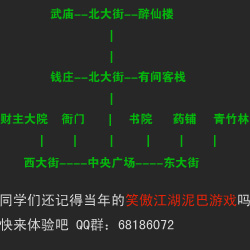# LPC基础数据类型:array和mapping

 [日期：2008-12-25] 来源：  作者：泥巴创世纪 [字体：大 中 小]

MudOS 提供了一种叫做 mapping 的数据类型，mapping

1、数据库
2、代替“struct”类型

mapping x;

x = ([ key0 : value0, key1 : value1, ... ]);

(注意：“x = ([]);”可用于创建一个空的 mapping)

type”这样的出错信息。

x[key] = value;

“x”的 mapping，如果这个 mapping 里面已经有了这个键，

(动态的)分配出来，然后这个 (key, value) 的对照将被插

write(x[key] + "\n");

map_delete(x, key);

undefinedp(x[key])

if (undefinedp(value = x["MudOS"]))
{
write("“MudOS”并未在映射“x”中用作一个"
"键。\n");
} else
{
write("“MudOS”键的值是：" + value + "。"
"\n");
}

mixed *idx;
mapping x;

x = ([ "x" : 3, "y" : 4]);
idx = keys(x);

idx == ({ "x", "y" }) 或 ({ "y", "x" })。

idx = values(x);

(0, 0)。each() 返回的顺序是和 key()、values() 的顺序

mixed *pair;

while ((pair = each(x)) != ({ }))
{
write("key   = " + pair + "\n");
write("value = " + pair + "\n");
}

(注：MudOS v22 中 each() 函数已经被去掉)。

mapping x, y;

x = ([ ]);
y = ([ ]);

y["a"] = "c";
x["b"] = y;

mapping r1, r2, a;

r1 = ([ ]);
r2 = ([ ]);

r1["driver"] = "mudlib";
r2["mudlib"] = "castle";

a = r1 * r2

a = r1 + r2

a["driver"] == "mudlib" 而且 a["mudlib"] == "castle"

+= 运算符也是支持的，因此你可以这样用：

a += ([ key : value ]);

a[key] = value;

sizeof() 这个 efun 可以用于测定这个 mapping 中一共有

write("映射“x”包括" + sizeof(x) + "个元素"
"。\n");

MudOS 的 mapping是使用一个可扩展的哈希表来实现的，这

MudOS 的 mapping类型最初由 Whiplash@TMI 完成，一部分

associative   ：联合
key         ：键
dynamically   ：动态
mapping       ：映射
implementation ：执行

 12下一页

52mud提供